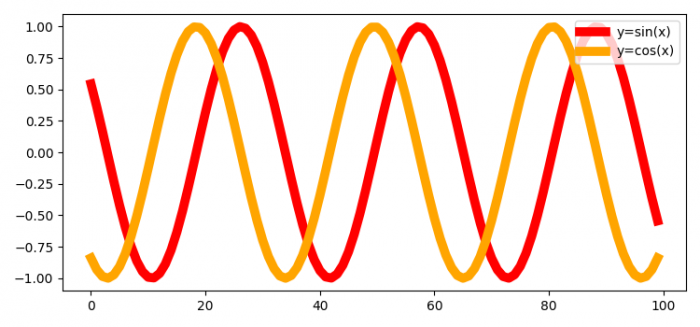# How to get all the legends from a plot in Matplotlib?

To get all the legends from a plot in matplotlib, we can use the get_children() method to get all the properties of an axis, then iterate all the properties. If an item is an instance of a Legend, then get the legend texts.

## steps

• Set the figure size and adjust the padding between and around the subplots.

• Create x data points using numpy.

• Create a figure and a set of subplots.

• Plot sin(x) and cos(x) using plot() method with different labels and colors.

• Get the children of the axis and get the texts of the legend.

• To display the figure, use show() method.

## Example

import numpy as np
from matplotlib import pyplot as plt
import matplotlib

plt.rcParams["figure.figsize"] = [7.50, 3.50]
plt.rcParams["figure.autolayout"] = True

x = np.linspace(-10, 10, 100)
fig, ax = plt.subplots()

ax.plot(np.sin(x), color='red', lw=7, label="y=sin(x)")
ax.plot(np.cos(x), color='orange', lw=7, label="y=cos(x)")

plt.legend(loc='upper right')

for item in ax.get_children():
if isinstance(item, matplotlib.legend.Legend):
print(item.texts)

plt.show()

## Output